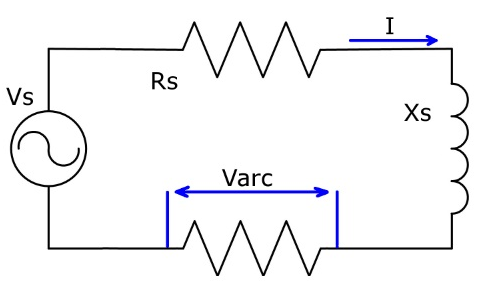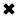Single Phase Arc Flash Calculations ExplainedWorker attired in PPE to safeguard against the arc-flash.

The electric arc flash hazard from alternating-current (AC) 3-phase (3Φ) power systems has been well documented due to the research by many scientists and the introduction of IEEE 1584 Guide for Arc Flash Hazard Calculations. Yet the arc flash hazard is not limited solely to AC 3Φ power systems. A notable hazard can also exist within single-phase (1Φ) systems, however AC 1Φ arc flash equations and calculation methods are not yet part of any standard. The paper proposes a comprehensive method for addressing 1Φ arc flash calculations that may be considered at least until an official standard for single-phase arc flash calculations has been released.

www.electricityforum.com/electrical-training/arc-flash-training

ARCING CURRENT

Votage drop Varc across the equivalent arc resistance which is introduced by the arc plasma in typical AC circuit depicted on Figure 1 can be expressed as:

Varc = -I * Rs + (Vs2 - I2*Xs2)1/2                                                               Equation 1

where I is current in Amps, Vs stands for an open source voltage in Volts, Rs and Xs are system resistance and reactance respectively, including source and feeders.Figure 1. Single & three phase AC arc flash equivalent circuit.

It was demonstrated that there is a minimum voltage needed to maintain an arc. That minimum depends on the current magnitude, gap width, and orientation of the electrodes. This transitional point can be calculated by :

It = 10 + 0.2 * G                                                                                          Equation 2

where
G - conductor gap distance in millimeters
It - the transitional point current measured in amperes

Above that minimum, the arc V-I characteristic can be expressed as :

Varc = (20 + 0.534 * G) * Iarc0.12                                                                       Equation 3

or alternatively as:

Iarc = [Varc / (20 + 0.534 * G)]8.33                                                       Equation 4

where
Varc - arcing voltage in Volts
Iarc - arcing current in Amps

Equations (1) through (3) can be solved for the maximum conductor gap where the arc can likely be sustained under the specified system voltage, the equivalent source X/R ratio and available short circuit fault current.

To find the point where the arc V-I characteristic crosses the circuit load line, solve equations (1) and (4) using the iterative method. As the first approximation, assume Iarc is equal to half of the available short circuit fault current. Then, follow the steps below:

1. determine Varc from equation (1)
2. substitute Varc into equation (4) to determine new Iarc

Cycle through the steps 1 and 2 above until the answers for Varc converge.

Table 1 shows arcing current values measured by two laboratories  along with accompanying system parameters, and the current values calculated solving equations 1 through 4 using the same parameters. Calculated arcing current values  nearly match the actual test data.

Table 1 - A comparison between actual arcing current data and the output of ArcMaster software

POWER AND ENERGY IN THE ARC

The energy released during an arc flash event is roughly proportional to the arc duration. The upstream protective device operation controls the arc flash duration. A fuse or properly maintained over-current protection device has a predictable time to open the circuit with a specific arc current value. Hence, arcing current impacts the released energy directly through the current itself and through interaction with the protection device.

The power in the arc can be calculated by:

Parc = Iarc * Varc                                                                                        Equation 7

where the Parc is measured in watts.

The energy in the arc is a function of power and time:

Earc = Parc * tarc                                                                                        Equation 8

where the Earc is measured in Joules.

Incident energy exposure for an open-air arc where the heat transfer depends on the spherical energy density is then expressed as:

Eiair = Earc/(4 * π * D2)                                                                            Equation 9

where
Eiair - incident energy from an open air arc at distance D in Joules/cm2
D - distance from the arc in centimeters

This formula assumes the radiant heat transfer. Not all of the arc energy will be transferred as radiant heat as a portion of the energy is transformed into pressure rise and in heat conducted to the electrode material. Therefore, the equation (9) will produce a conservative but safe estimate of incident energy exposure.

For the arc in a box, the enclosure has a focusing effect on the incident energy. For the selected enclosure type and test distance, the incident energy from an arc flash in a box can be calculated by :

Eibox = K * Earc / (A2 + D2)                                                                     Equation 10

where
Eibox - the incident energy from an arc flash in a box in Joules/cm2
D - distance from the arc in centimeters

A and K are obtained from optimal values listed in table below:

ARC FLASH BOUNDARY

Equation (9) written in terms of arc flash boundary, becomes:

AFB = [Earc / (4 * π * Et)]0.5                                                                    Equation 11

where
AFB - arc flash boundary measured in centimeters
Et - threshold incident energy to second degree burn in J/cm2 calculated by [4, 6]:

Et = 5 * t0.3                                                                                                       Equation 12

where t stands for exposure time in seconds.

Likewise, equation (10) written in terms of arc flash boundary, becomes:

AFB = [K * Earc / Et - A2]0.5                                                                     Equation 13

CONCLUSION

A semi-empirical model of single phase arc flash hazard has been develope. The maximum conductor gap where the arc can likely be sustained under the specified system voltage, the equivalent source X/R ratio and available short circuit fault current was derived from the analytical expressions for the transitional point current, AC arc flash non-linear load line and the arc V-I characteristic. Arcing current has been determined iteratively using the arc V-I characteristic and load line. The calculated arcing current values are very close to the actual test data. Wilkins optimal values were applied in determining the incident energy for arc in a box and the arc flash boundary was calculated based on the variable threshold incident energy to second degree burn. The proposed model assumes all the energy generated by an arc is converted into the radiated heat resulting in conservative but safe estimate of arc flash boundary. One day single-phase AC arc flash calculations will be part of an official standard but until then this is one method for addressing single-phase AC arc flash calculations.

References

1. A.D. Stokes and W.T. Oppenlander, "Electric arcs in open air", Journal of Physics D: Applied Physics, 1991, pp. 26-35
2. L. Fisher, "Resistance of Low-Voltage AC Arcs", IEEE Transactions on Industry and General Applications, vol. IGA-6, No.6, Nov/Dec 1970
3. R. Wilkins, "Simple improved equations for arc flash hazard analysis", IEEE Electrical Safety Forum, August 2004
4. M. Furtak and L. Silecky, "Evaluation of onset to second degree burn energy in arc flash hazard analysis", IAEI News, July-August 2012
6. ASTM F1959/F1959M, “Standard Test Method for Determining the Arc Rating of Materials for Clothing”, ASTM International, 1959